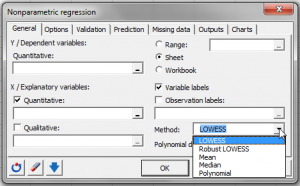# Nonparametric regression (Kernel and Lowess)

Nonparametric regression is used for prediction and is reliable even if hypotheses of linear regression are not verified. Do it in Excel with the XLSTAT software.## When to use nonparametric regression

Nonparametric regression can be used when the hypotheses about more classical regression methods, such as linear regression, cannot be verified or when we are mainly interested in only the predictive quality of the model and not its structure.

## Nonparametric regression in XLSTAT

XLSTAT offers two types of nonparametric regressions: Kernel and Lowess.

### Kernel regression

Kernel regression is a modeling tool which belongs to the family of smoothing methods. Unlike linear regression which is both used to explain phenomena and for prediction (understanding a phenomenon to be able to predict it afterwards), Kernel regression is mostly used for prediction. The structure of the model is variable and complex, the latter working like a filter or black box. There are many variations of Kernel regression in existence.

As with any modeling method, a learning sample of size nlearn is used to estimate the parameters of the model. A sample of size nvalid can then be used to evaluate the quality of the model. Lastly, the model can be applied to a prediction sample of size npred, for which the values of the dependent variable Y are unknown.

The characteristics of Kernel Regression are:

1. The use of a kernel function, to weigh the observations of the learning sample, depending on their "distance" from the predicted observation.

The kernel functions available in XLSTAT are:

• Uniform
• Triangle
• Epanechnikov
• Quartic
• Triweight
• Tricube
• Gaussian
• Cosine
2. The bandwidth associated to each variable. It is involved in calculating the kernel and the weights of the observations, and differentiates or rescales the relative weights of the variables while at the same time reducing or augmenting the impact of observations of the learning sample, depending on how far they are from the observation to predict.
3. The polynomial degree used when fitting the model to the observations of the learning sample. Two strategies are suggested in order to restrict the size of the learning sample taken into account for the estimation of the parameters of the polynomial: Moving window and k nearest neighbors.

### LOWESS regression

Locally weighted regression and smoothing scatter plots or LOWESS regression was introduced to create smooth curves through scattergrams.

LOWESS regression is very similar to Kernel regression as it is also based on polynomial regression and requires a kernel function to weight the observations.

## Results for nonparametric regression in XLSTAT

• Descriptive statistics: The table of descriptive statistics shows the simple statistics for all the variables selected. The number of missing values, the number of non-missing values, the mean and the standard deviation (unbiased) are displayed for the quantitative variables. For qualitative variables, including the dependent variable, the categories with their respective frequencies and percentages are displayed.
• Correlation matrix: This table displays the correlations between the selected variables.
• Goodness of fit coefficients: This table shows the following statistics:
• The determination coefficient R2;
• The sum of squares of the errors (or residuals) of the model (SSE or SSR respectively);
• The means of the squares of the errors (or residuals) of the model (MSE or MSR);
• The root mean squares of the errors (or residuals) of the model (RMSE or RMSR).
• Predictions and residuals: Table giving for each observation the input data, the value predicted by the model and the residuals.

## Charts for nonparametric regression in XLSTAT

If only one quantitative explanatory variable or temporal variable has been selected as a function of time, the first chart shows the data and the curve for the predictions made by the model. If there are several explanatory variables, the first chart shows the observed data and predictions as a function of the first explanatory variable selected.

The second chart displayed is the bar chart of the residuals.### analyze your data with xlstat

14-day free trial

Included in

Related features